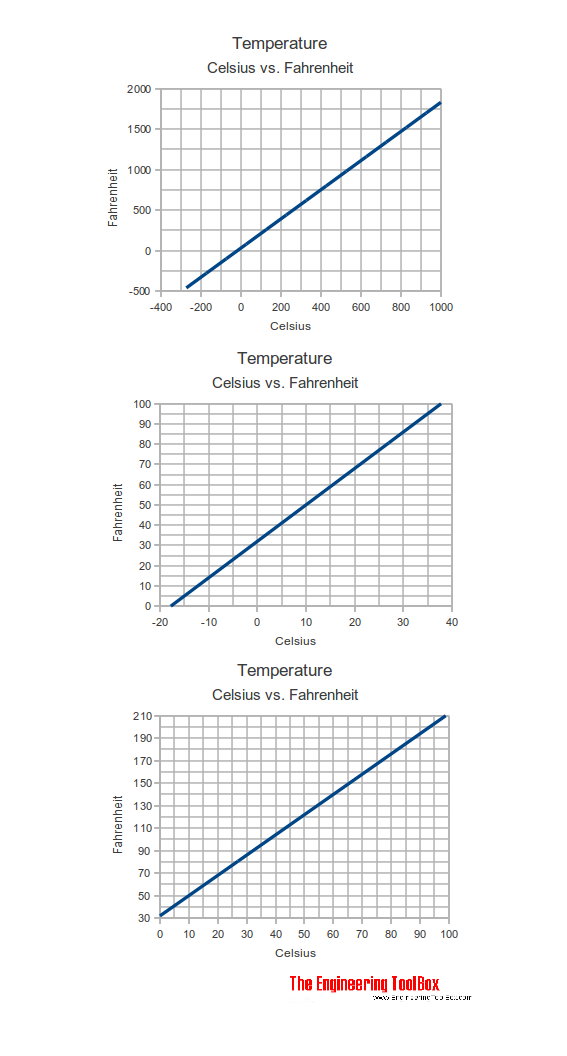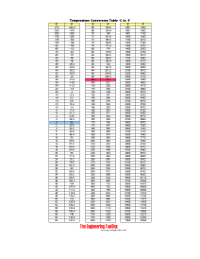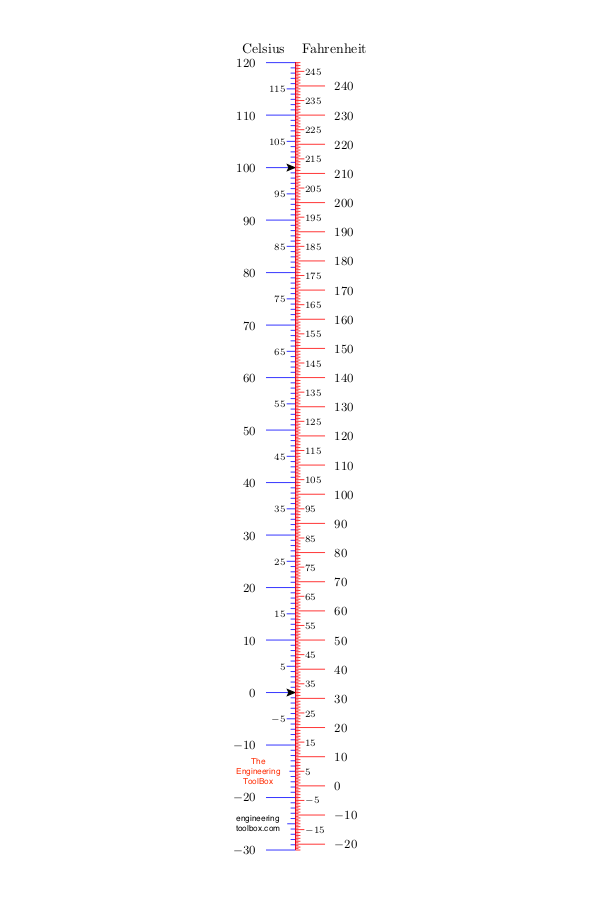Engineering ToolBox - Resources, Tools and Basic Information for Engineering and Design of Technical Applications!

# Temperature

## Introduction to temperature - including Celsius, Fahrenheit, Kelvin and Rankine definitions - and an online temperature converter.

Temperature (sometimes called thermodynamic temperature) is a measure of the average kinetic energy of the particles in a system. Temperature is the degree of "hotness" ( or "coldness") - a measure of the heat intensity. The most common symbol or abbreviation for temperature is T.

When two objects of different temperatures are in contact - the warmer object becomes colder while the colder object becomes warmer. This means that heat flows from the warmer to the colder object.

### Temperature Converter

Convert between °C (Celsius), °F (Fahrenheit), K (Kelvin) and °R (Rankine) with the calculator below:

Temperature

°C

°F

K

°R

 Rankine Kelvin Fahrenheit Celsius °R K °F C 0 0 -459.67 -273.15 100 55.56 -359.67 -217.59 180 100 -279.67 -173.15 459.67 255.37 0 -17.78 491.67 273.15 32 0 559.67 310.93 100 37.78 671.67 373.15 212 100

### Temperature Converter - Web App

Add the Temperature Converter Web App to your mobile device or desktop. The App is saved in your browser and works offline automatically after first visit.

### Degree Celsius (°C) and Degree Fahrenheit (°F)

A thermometer can help us determine how cold or hot a substance is. Temperature is in most of the world measured and reported in degrees Celsius (°C). In the U.S. it is common to report temperature in degrees Fahrenheit (°F). In the Celsius and Fahrenheit scales the temperatures where ice melts (water freezes) and water boils are used as reference points.

• In the Celsius scale the freezing point of water is defined as °C and the boiling point is defined as 100 °C
• In the Fahrenheit scale the water freezes at 32 °F and boils at 212 °F

In the Celsius scale there are 100 degrees between the freezing point and the boiling point of water compared to 180 degrees in the Fahrenheit scale. This means that °C = 1.8 °F (check the section about temperature differences below).

Values can be converted between the two temperature units by using the equations:

T(°F) = 1.8 T(°C) + 32                           (1)

T(°C) = (T(°F) - 32)/1.8                         (2)

where

T(°C)= temperature (°C )

T(°F)= temperature (°F)### Celsius vs. Fahrenheit

Temperature
oCoF
-20 -4
-15 5
-10 14
-5 23
0 32
5 41
10 50
15 59
20 68
25 77
30 86
35 95
40 104
45 113
50 122

Example: A patient with SARS (Severe Acute Respiratory Syndrome) has a temperature of 106 °F. What is the temperature in Celsius?

T(°C) = (106 °F - 32)/1.8   = 41.1 °C

### Temperature Conversion Table - oC vs °F### Temperature Difference - or Temperature Change - degree Celsius versus degree Fahrenheit

Note that for temperature difference (change) - as used in heat loss diagrams

• 1 degree Celsius of temperature difference equals 1.8 degree Fahrenheit of temperature difference

ΔT(°C) = ΔT(°F) / 1.8                            (3)

ΔT(°F) = 1.8 ΔT(°C)                            (4)

where

ΔT(°F) = temperature difference (°F)

ΔT(°C) = temperature difference (°C)

Example: Water is cooled from 100°C to 60°C. What is the temperature difference in °F?

Temperature difference in degrees Celsius:

ΔT(°C) = 100 °C - 60 °C = 40 C°

Note that °C is used for actual temperatures (temperature relative to 273.15 K) and C° is used for temperature differences.

Temperature difference in degrees Fahrenheit calculated by using (1)

100 °C = 1.8 (100 °C) + 32 = 212 °F

60 °C = 1.8 (60 °C) + 32 = 140 °F

ΔT(°F) =  212°F140°F = 72 °F

Temperature difference in degrees Fahrenheit calculated by using (3)

ΔT(°F) = 1.8 (40 C°) = 72 °F

#### Temperature Difference Converter

Temperature Difference

°F

### Kelvin - K

Another scale (common in science) is Kelvin, or the Absolute Temperature Scale. On the Kelvin scale the coldest temperature possible, -273 °C, has a value of 0 Kelvin (0 K) and is called the absolute zero. Units on the Kelvin scale are called Kelvins (K) and no degree symbol is used.
Because there are no lower temperatures than 0 K  - the Kelvin scale does not have negative numbers.

The Kelvin has the same incremental scale as the Celsius scale and one unit Kelvin is equal in size to one unit Celsius:

1 unit Kelvin  = 1 unit °C

ΔT(°K) = ΔT(°C)                                (5)

To calculate a Kelvin temperature, add 273 to the Celsius temperature:

T(K) = T(°C) + 273.15                        (6)

Example: What is the normal body temperature of 37 oC in the Kelvin scale?

T(K) = T(°C) + 273.15 = 37°C + 273.15 = 310.15 K

### Degree Rankine - R

In the English system the absolute temperature is in degrees Rankine (R), not in Fahrenheit:

T(°R) = 1.8*T(K)                                 (7)

T(°R) = 1.8*(T(°C)+273.15)

T(°R) = T(°F) + 459.67                      (8)

ΔT(°R) = ΔT(°F)                                (9)

### Celcius to Fahrenheit Converter (and vice versa)## Related Topics

### • Basics

The SI-system, unit converters, physical constants, drawing scales and more.

### • Temperature Measurement

Measurment of temperature - probes, sensors and transmitters.

### • Thermodynamics

Work, heat and energy systems.

## Related Documents

### Liquids and Gases - Boiling Points

Boiling temperatures for common liquids and gases - acetone, butane, propane and more.

### The Ideal Gas Law

The relationship between volume, pressure, temperature and quantity of a gas, including definition of gas density.

## Engineering ToolBox - SketchUp Extension - Online 3D modeling!

Add standard and customized parametric components - like flange beams, lumbers, piping, stairs and more - to your Sketchup model with the Engineering ToolBox - SketchUp Extension - enabled for use with the amazing, fun and free SketchUp Make and SketchUp Pro . Add the Engineering ToolBox extension to your SketchUp from the SketchUp Pro Sketchup Extension Warehouse!

Translate

## Privacy

We don't collect information from our users. Only emails and answers are saved in our archive. Cookies are only used in the browser to improve user experience.

Some of our calculators and applications let you save application data to your local computer. These applications will - due to browser restrictions - send data between your browser and our server. We don't save this data.